Ex 9.3

Chapter 9 Class 8 Mensuration
Serial order wise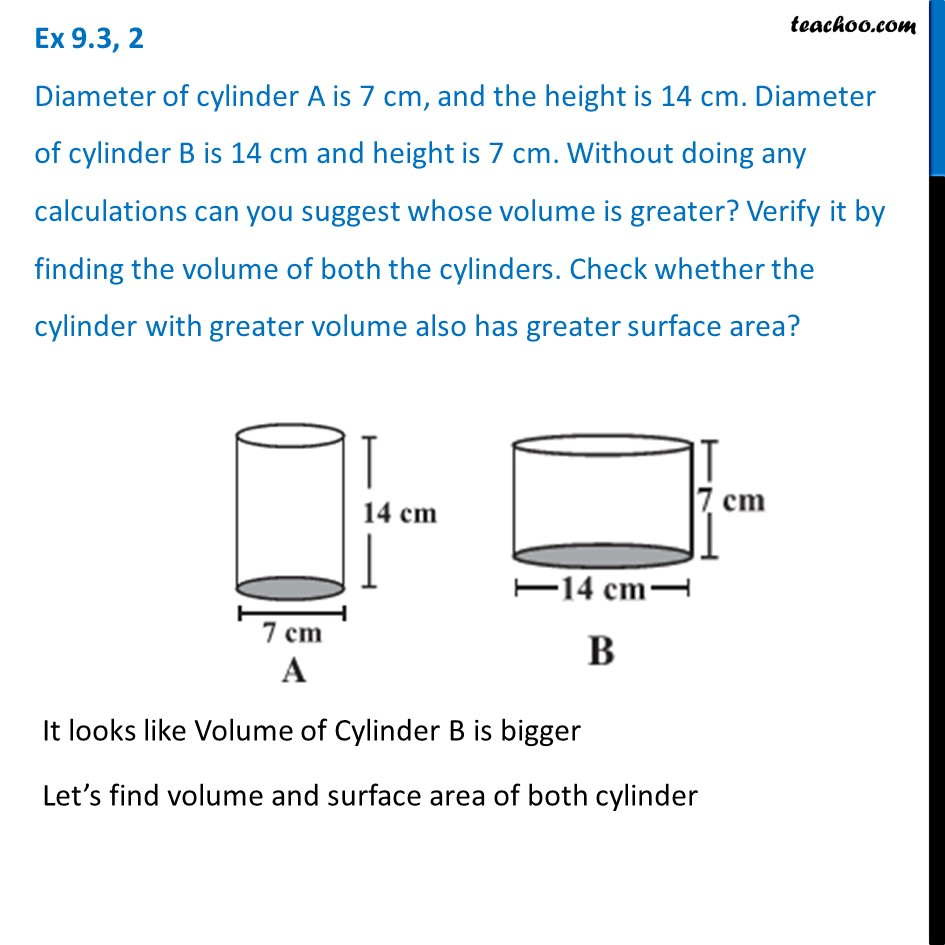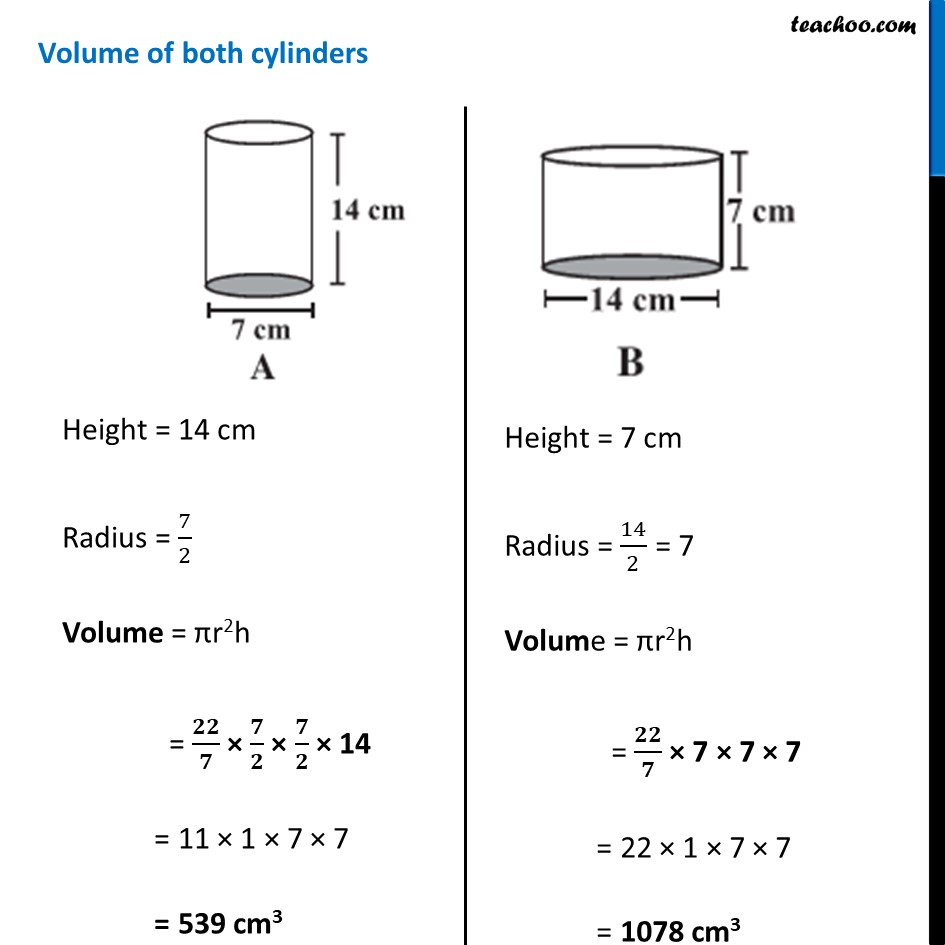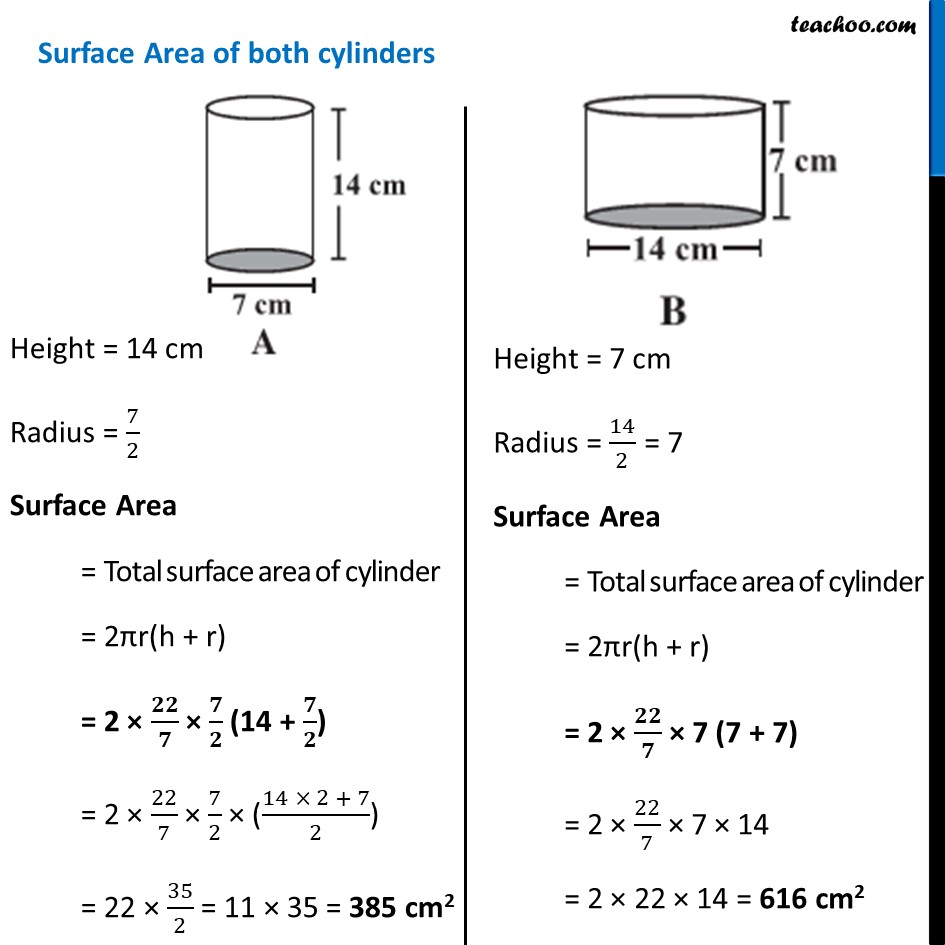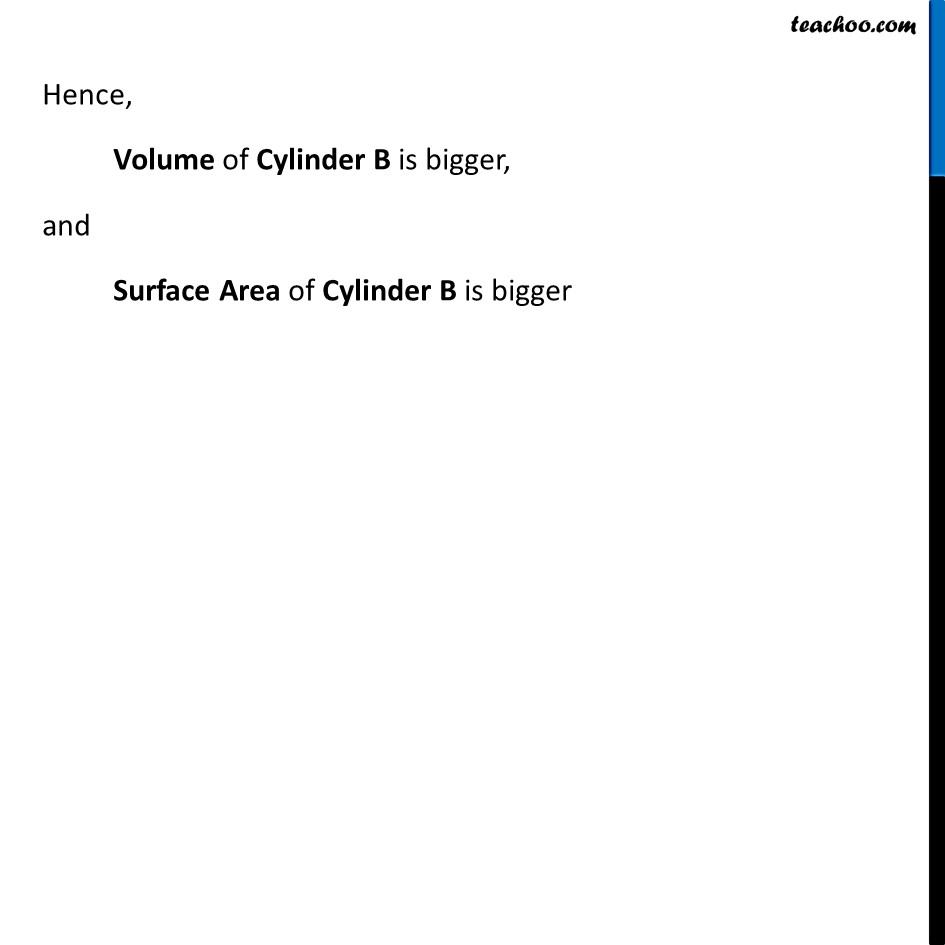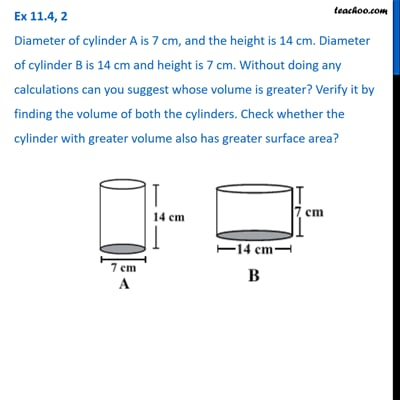This video is only available for Teachoo black users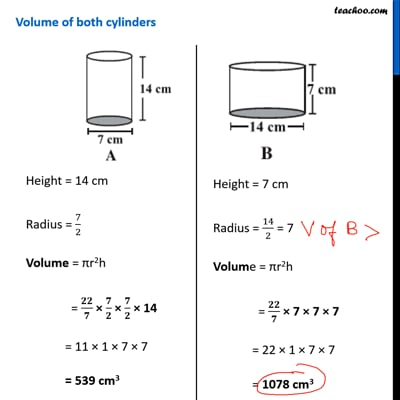This video is only available for Teachoo black users

Learn in your speed, with individual attention - Teachoo Maths 1-on-1 Class

### Transcript

Ex 9.3, 2 Diameter of cylinder A is 7 cm, and the height is 14 cm. Diameter of cylinder B is 14 cm and height is 7 cm. Without doing any calculations can you suggest whose volume is greater? Verify it by finding the volume of both the cylinders. Check whether the cylinder with greater volume also has greater surface area? It looks like Volume of Cylinder B is bigger Let’s find volume and surface area of both cylinder Height = 14 cm Radius = 7/2 Volume = πr2h = 𝟐𝟐/𝟕 × 𝟕/𝟐 × 𝟕/𝟐 × 14 = 11 × 1 × 7 × 7 = 539 cm3 Height = 7 cm Radius = 14/2 = 7 Volume = πr2h = 𝟐𝟐/𝟕 × 7 × 7 × 7 = 22 × 1 × 7 × 7 = 1078 cm3 Height = 14 cm Radius = 7/2 Surface Area = Total surface area of cylinder = 2πr(h + r) = 2 × 𝟐𝟐/𝟕 × 𝟕/𝟐 (14 + 𝟕/𝟐) = 2 × 22/7 × 7/2 × ((14 × 2 + 7)/2) = 22 × 35/2 = 11 × 35 = 385 cm2 Height = 7 cm Radius = 14/2 = 7 Surface Area = Total surface area of cylinder = 2πr(h + r) = 2 × 𝟐𝟐/𝟕 × 7 (7 + 7) = 2 × 22/7 × 7 × 14 = 2 × 22 × 14 = 616 cm2 Hence, Volume of Cylinder B is bigger, and Surface Area of Cylinder B is bigger